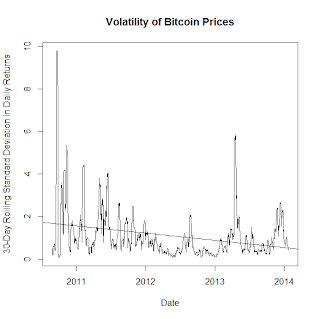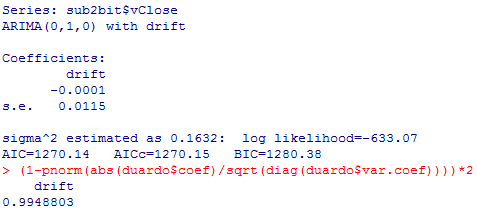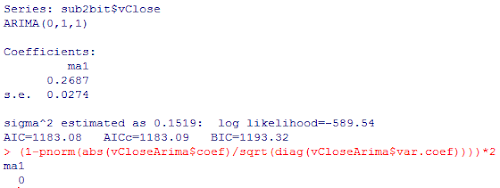### No evidence of declining volatility for Bitcoins

1/22/2014 10:43:00 PM
Tweetable

Now here's Robert Sams, in rebuttal:
"But the claim that “there is a clear trend of falling volatility over time” isn’t defensible at all."
Sams replicated Dourado's results, and then did his own analysis to suggest that Dourado's regression wasn't valid. In particular, Sams ran essentially the same regression, but on a rolling 2-year window to show how the coefficient estimate is evolving over time as new data comes in:Sams's results, showing that the OLS coefficient is not a consistent estimator.Sams adds:
"I don’t like using trend lines in analysing financial timeseries."
No, I suppose I don't like that either.

Listen, we have a term for time series whose time-trends shift over time--it's "non-stationary." How did two economists do regressions on a time series, and yet no one has 1)tested for stationarity or 2)done any time-series econometrics? We have tools for these kinds of questions!

Ok, now that I'm done snarking, I will say that Sams is exactly correct, if a little imprecise. Dourado's error is in assuming that the series he was regressing was stationary when such an assumption cannot be supported. Like Sams, I have also replicated Dourado's results:My replication of Dourado's results.And here's my code in R (you need to have the TTR library installed):Now, the problem with Dourado's model is that the data aren't stationary, so his coefficient is not a consistent estimator of the true time trend. It turns out that there's a clever little function on R to test this:That tells us we need to difference the volatility series before we can say anything about the trends. If your curious, the data failed the Kwiatkowski–Phillips–Schmidt–Shin test, though not the Augmented Dickey-Fuller test for a unit root.

Ok, let's look at this from a different angle. A more precise description of Dourado's hypothesis is that the time-trend in question has a below-zero drift term in the data-generating process. Well, here's that:In principle, the drift coefficient here should be identical to Dourado's coefficient. Yet, because this series is stationary and Dourado's isn't, we can now see that the coefficient is not statistically significant at all. I've calculated a p-value though it's not part of R's standard output--from the looks of it, the probability is quite low that the time trend isn't actually zero.Of course, since this is a likelihood estimation, model selection should really be based on one or another information criterion, not on individual p-values. So just for kicks, here's the (stationary) time-series model that minimizes the Bayesian-schwarz information criterion:What actual time-series econometrics looks like.Oh, look at that--no drift term!

The bottom line here is that Dourado aint got it. I cannot tell you which way Bitcoin volatility is going, but I can tell you that Dourado can't either.

Full R code below: